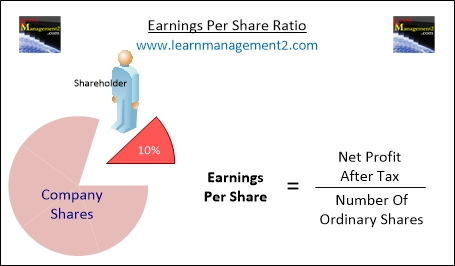# Earnings Per Share Ratio

Anybody that buys shares in a business is known as a shareholder. Shareholder ratios calculate the return shareholders receive from the money they have invested in a firm; in this article we will discuss the shareholder ratio called The Earnings Per Share Ratio.

Click to learn about the Price Earnings Ratio and The Dividend Yield, the other shareholder ratios.Diagram showing how to calculate Earnings Per Share

### Calculating Earnings Per Share

To calculate the earnings per share you will need to find out the number of ordinary shares that have been issued and the net profit after tax for the firm. Divide the net profit after tax by the number of ordinary shares to obtain the earnings per share.

Earnings per                Net profit after tax
Share              =          _________

Number of ordinary shares

### Example Calculation

For example if a firm’s net profit after tax is £10000 and they have 20000 ordinary shareholders earnings per share would be calculated as follows:

Earnings per                £10000
Share              =          _________      =          £0.50

20000

As earnings per share are usually expressed in pence in our example the earnings per share is 50p

### Conclusion

We hope you have enjoyed learning about the Earnings Per Share Ratio, if you would like to learn more about shareholder ratios, click to learn about the Price-Earnings Ratio and The Dividend Yield the other shareholder ratios.